JEE  >  JEE Main Physics Mock - 1

# JEE Main Physics Mock - 1

Test Description

## 25 Questions MCQ Test JEE Main & Advanced Mock Test Series | JEE Main Physics Mock - 1

JEE Main Physics Mock - 1 for JEE 2023 is part of JEE Main & Advanced Mock Test Series preparation. The JEE Main Physics Mock - 1 questions and answers have been prepared according to the JEE exam syllabus.The JEE Main Physics Mock - 1 MCQs are made for JEE 2023 Exam. Find important definitions, questions, notes, meanings, examples, exercises, MCQs and online tests for JEE Main Physics Mock - 1 below.
Solutions of JEE Main Physics Mock - 1 questions in English are available as part of our JEE Main & Advanced Mock Test Series for JEE & JEE Main Physics Mock - 1 solutions in Hindi for JEE Main & Advanced Mock Test Series course. Download more important topics, notes, lectures and mock test series for JEE Exam by signing up for free. Attempt JEE Main Physics Mock - 1 | 25 questions in 60 minutes | Mock test for JEE preparation | Free important questions MCQ to study JEE Main & Advanced Mock Test Series for JEE Exam | Download free PDF with solutions
 1 Crore+ students have signed up on EduRev. Have you?
JEE Main Physics Mock - 1 - Question 1

### An electric lamp is connected to 220 V, 50 Hz supply. Then the peak value of voltage is

Detailed Solution for JEE Main Physics Mock - 1 - Question 1

Solution :- V(0) = Vrms × 2

= 220 × √2

= 310

JEE Main Physics Mock - 1 - Question 2

### In a step-up transformer the voltage in the primary is 220 V and the current is 5A. The secondary voltage is found to be 22000V. The current in the secondary (neglect losses) is

Detailed Solution for JEE Main Physics Mock - 1 - Question 2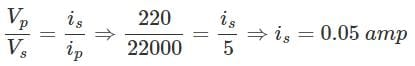JEE Main Physics Mock - 1 - Question 3

### A body moves along a circular path of radius 10 m and the coefficient of friction is 0.5. What should be the angular velocity of the body, if it is not to slip on the surface?

Detailed Solution for JEE Main Physics Mock - 1 - Question 3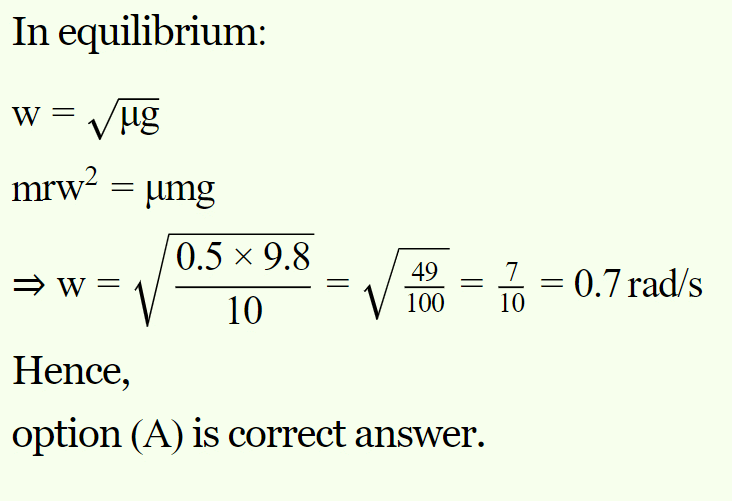JEE Main Physics Mock - 1 - Question 4

K.E. of emitted cathode rays is dependent on

Detailed Solution for JEE Main Physics Mock - 1 - Question 4

Higher the voltage, higher is the KE.  Higher the work function, smaller is the KE.

JEE Main Physics Mock - 1 - Question 5

A semi circle arc of radius 'a' is charged uniformly and the charge per unit length is λ. The electric field at its centre is

Detailed Solution for JEE Main Physics Mock - 1 - Question 5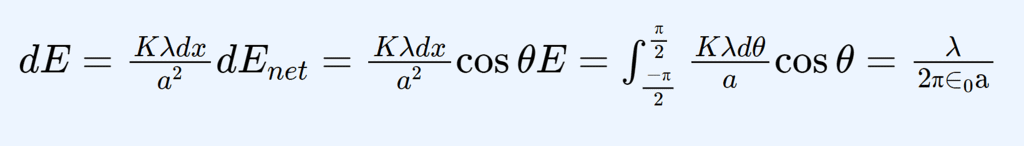JEE Main Physics Mock - 1 - Question 6

A gun fires the bullets each of mass 10 g with a velocity of 50 m−s-1. If 1000 bullets are fired per second, then thrust on the shoulder will be

Detailed Solution for JEE Main Physics Mock - 1 - Question 6

Mass of bullet

m=0.01Kg

v=50m/s

n=1000

Force on the man is equal to the change in momentum of the bullets coming out of the gun

F = mvn/t​

F=(0.01×50×1000)​/1

Therefore, F = 500N

JEE Main Physics Mock - 1 - Question 7

An electric dipole is placed along the X-axis at the origin O. A point P is at a distance of 20 cm from this origin such that OP makes an angle π/3 with the X axis. If electric field at P makes an angle θ with X-axis, the value of θ is

Detailed Solution for JEE Main Physics Mock - 1 - Question 7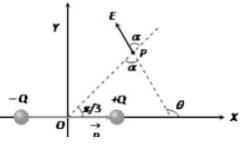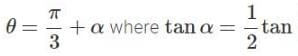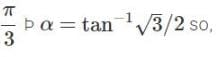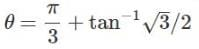JEE Main Physics Mock - 1 - Question 8

A metre stick AB hinged (without friction) at the end A as shown in the figure is kept horizontal by means of a string tied to the end B, the other end of the string being tied to a hook. The string is carefully cut (or burnt), and the scale executes angular oscillations about an axis passing through the end A. What is the speed of the end B when the metre stick assumes vertical position immediately after the string is burnt? (Acceleration due to gravity = 10 ms–2)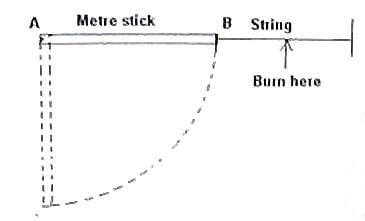Detailed Solution for JEE Main Physics Mock - 1 - Question 8The centre of gravity of the metre stick is at its middle. When the string is burnt, the height of the centre of gravity is reduced by 0.5 m, thereby reducing the gravitational potential energy of the metre stick by MghM×10×0.5 = 5joule, where M is the mass of the metre stick.

The decrease in the potential energy is equal to the increase in kinetic energy. Therefore in the vertical position of the metre stick, its kinetic energy

(½)Iω2 = 5M

Since the moment of inertia of a uniform bar of length about a normal axis through ite mid point is ML2/12, its moment of inertia about a parallel axis through its end is ML2/3. [You will get this by applying the parallel axis theorem (see the post dated 2oth January 2008)]

Therefore, we have (½)(ML2/3)ω2 = 5M

Since ω = v/L and L = 1 metre, the above equation becomes

v2/6 = 5, from which = √30 = 5.4 ms–1 (approximately).

JEE Main Physics Mock - 1 - Question 9

The symbolic representation of four logic gates are given below: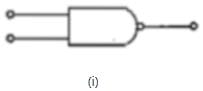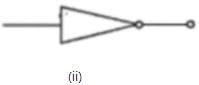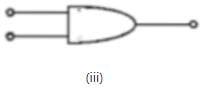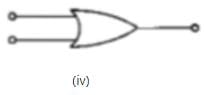The logic symbols for OR, NOT and NAND gates are respectively:

Detailed Solution for JEE Main Physics Mock - 1 - Question 9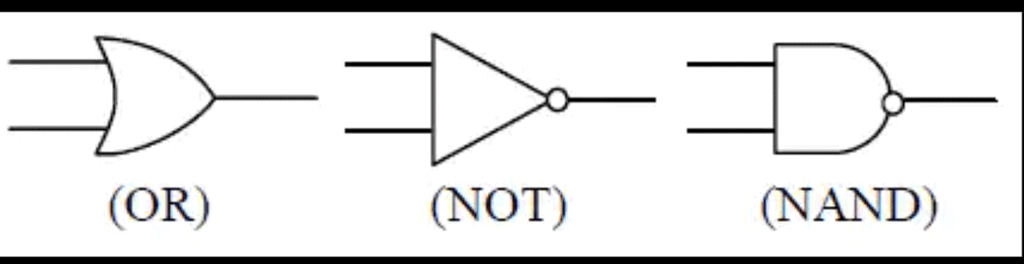JEE Main Physics Mock - 1 - Question 10

In a capillary tube, water rises upto 3 mm. The height of water that will rise in another capillary tube having one-third radius of the first is

Detailed Solution for JEE Main Physics Mock - 1 - Question 10

The narrower the tube, the higher is the rise in water.

When a glass capillary

h=2Tcosθ​/Rpg

h∝1/R​

h2/​h1​​=R1/​R2​​

​3/h2​=1/3​

h2​=9mm

JEE Main Physics Mock - 1 - Question 11

If a long hollow copper pipe carries a current then magnetic field produced will be

Detailed Solution for JEE Main Physics Mock - 1 - Question 11JEE Main Physics Mock - 1 - Question 12

A chain reaction in fission of uranium is possible because

Detailed Solution for JEE Main Physics Mock - 1 - Question 12

A nuclear chain reaction occurs when one single nuclear reaction causes an average of one or more subsequent nuclear reactions, thus leading to the possibility of a self-propagating series of these reactions.

JEE Main Physics Mock - 1 - Question 13

Two bodies of mass 10 kg and 5 kg moving in concentric orbits of radii R and r such that their periods are the same. Then the ratio between their centripetal acceleration is

Detailed Solution for JEE Main Physics Mock - 1 - Question 13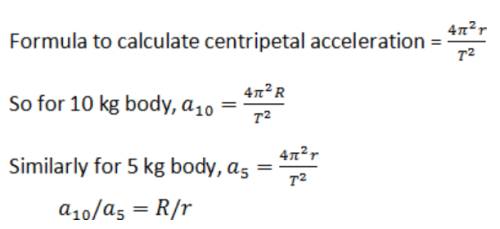JEE Main Physics Mock - 1 - Question 14

When there is an electric current through a conducting wire along its length then an electric field must exist

Detailed Solution for JEE Main Physics Mock - 1 - Question 14

As current is flowing through it , it means charges are flowing along its length therefore heir must be some electric field parallel to the length of the wire. Hence correct option is B.

JEE Main Physics Mock - 1 - Question 15

In the following question, a Statement of Assertion (A) is given followed by a corresponding Reason (R) just below it. Read the Statements carefully and mark the correct answer-
Reason(R):All the elements above lead are unstable

Detailed Solution for JEE Main Physics Mock - 1 - Question 15

For all 4n,4n+2 series elements, on emission of different kind of rays, they form different isotopes of lead and also all element above lead are unstable .
But, 4n+1 series elements (Neptunium series), end in ​Bi (Bismuth not lead)

JEE Main Physics Mock - 1 - Question 16

The moment of inertia of a uniform disc about an axis passing through its centre and perpendicular to its plane is 1kg - m2. It is rotating with an angular velocity 100 radians/second. Another identical disc is gently placed on it so that their centres coincide. Now these two discs together continue to rotate about the same axis. Then the loss in kinetic energy in kilo joules is

Detailed Solution for JEE Main Physics Mock - 1 - Question 16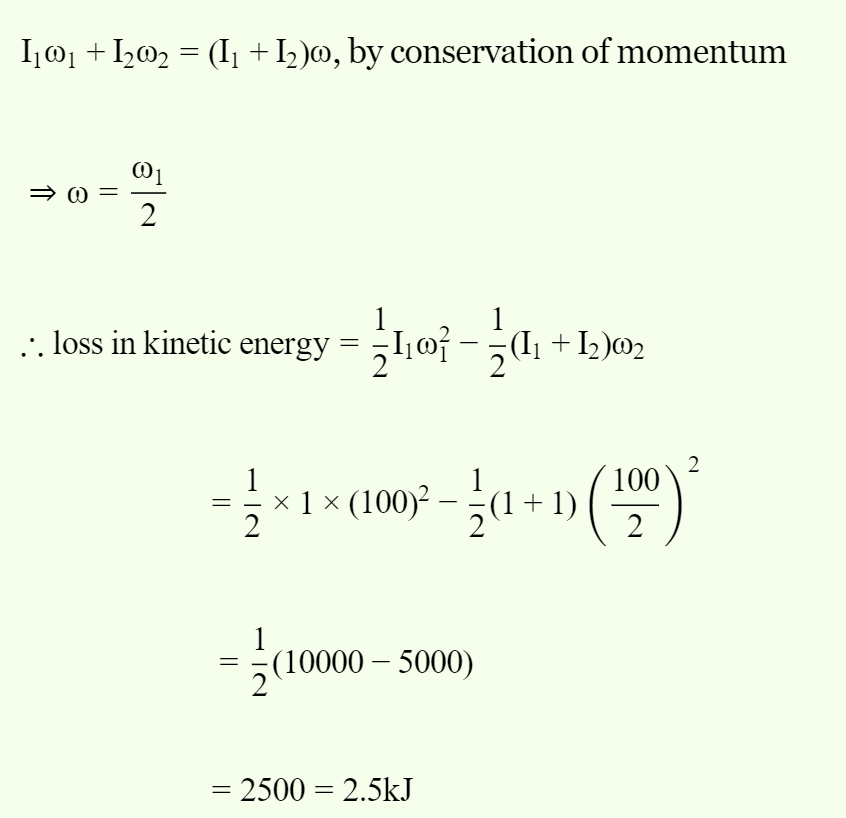JEE Main Physics Mock - 1 - Question 17

The internal energy of the gas increases when

Detailed Solution for JEE Main Physics Mock - 1 - Question 17

When a gas expands adiabatically, it loses internal energy equal to the work done by the gas. In this case the temperature of the gas decreases.

When a gas is compressed adiabatically, it gains internal energy equal to the work done on the gas. In this case the temperature of the gas increases.

JEE Main Physics Mock - 1 - Question 18

The dimensional equation for magnetic flux is

Detailed Solution for JEE Main Physics Mock - 1 - Question 18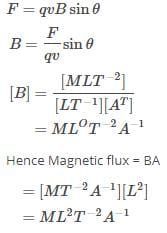JEE Main Physics Mock - 1 - Question 19

If T is the reverberation time of an auditorium of volume V, then

Detailed Solution for JEE Main Physics Mock - 1 - Question 19

Generally reverberation time is proportional to linear dimensions of the room. Hence, it will be proportional to the volume of the room.

JEE Main Physics Mock - 1 - Question 20

Consider the following statements.
(i) All isotopes of an element have the same number of neutrons.
(ii) Only one isotope of an element can be stable and non-radioactive.
(iii) All elements have isotopes.
(iv) All isotopes of Carbon can form chemical compounds with Oxygen-16.
The correct option regarding an isotope is?

Detailed Solution for JEE Main Physics Mock - 1 - Question 20

All elements have isotopes--atoms of the same element can have different number of neutrons.
Carbon form compounds (carbon monoxide and carbon dioxide) with oxygen. This means that all isotopes of carbon can form compounds (carbon monoxide and carbon dioxide) with all isotopes of oxygen, including oxygen-16.

*Answer can only contain numeric values
JEE Main Physics Mock - 1 - Question 21

The orbital velocity of an artificial satellite in a circular orbit just above the earth’s surface is v0. The orbital velocity of satellite orbiting at an altitude of double the radius of earth is V0/√n Then the value of n is :

Detailed Solution for JEE Main Physics Mock - 1 - Question 21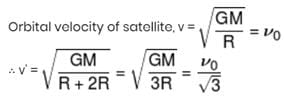*Answer can only contain numeric values
JEE Main Physics Mock - 1 - Question 22

A 300 W carrier is modulated to a depth 75%. The total power in the modulated wave is:-

Detailed Solution for JEE Main Physics Mock - 1 - Question 22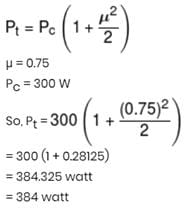*Answer can only contain numeric values
JEE Main Physics Mock - 1 - Question 23

A conducting rod of 1 m length and 1 kg mass is suspended by two vertical wires through its ends. An external magnetic field of 2T is applied normal to the rod. Now, the current to be passed through the rod so as to make the tension in the wires zero is : (Take g = 10 ms–2) :-

Detailed Solution for JEE Main Physics Mock - 1 - Question 23

Magnetic force on rod = BIℓ
Weight of the rod = mg
For no tension in wire, BIℓ = mg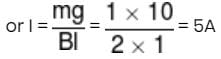*Answer can only contain numeric values
JEE Main Physics Mock - 1 - Question 24

At what speed a ball must be projected vertically upward so that distance travelled by it in 5th second is equal to distance travelled in sixth second (in m/s):-

Detailed Solution for JEE Main Physics Mock - 1 - Question 24

time of flight = (5 sec) × 2 = 10 sec
µ = gt = 9.8 × 5 = 49 m/s

*Answer can only contain numeric values
JEE Main Physics Mock - 1 - Question 25

In thermodynamic process pressure of a fixed mass of gas is changed in such a manner that the gas releases 30 joule of heat and 18 joule of work was done on the gas. If the initial internal energy of the gas was 60 joule, then, the final internal energy (in J) will be:

Detailed Solution for JEE Main Physics Mock - 1 - Question 25

ΔQ = –30J
Δw = –18
Ui = 60 J
Uf = ?
ΔQ = Δw + Uf – Ui
–30 = –18 + Uf – 60 ⇒ Uf = 48 J

## JEE Main & Advanced Mock Test Series

2 videos|325 docs|189 tests
 Use Code STAYHOME200 and get INR 200 additional OFF Use Coupon Code
Information about JEE Main Physics Mock - 1 Page
In this test you can find the Exam questions for JEE Main Physics Mock - 1 solved & explained in the simplest way possible. Besides giving Questions and answers for JEE Main Physics Mock - 1, EduRev gives you an ample number of Online tests for practice

## JEE Main & Advanced Mock Test Series

2 videos|325 docs|189 tests